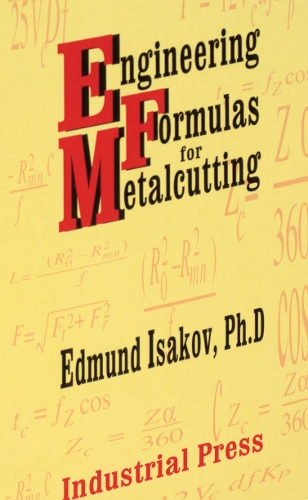•# Engineering Formulas for Metalcutting (Hardback)

(author)
£39.95
Hardback 240 Pages / Published: 01/01/2004
• We can order this

Usually dispatched within 3 weeks

Maximize the productivity of cutting.Linear regression equations for converting Rockwell, Vickers, Knoop, and Scleroscope hardness numbers into Brinell hardness numbers.Formulas and linear regression equations for calculating ultimate tensile strength of the most commonly used work materials in relationship with their hardness.Formulas for calculating the number of inserts simultaneously engaged with the workpiece depending on milling conditions.Formulas to calculate machining time when facing, cutoff, and deep grooving and for feed and radial forces in relationship with tangential force.Set of formulas to calculate overhang of boring bars made of tungsten heavy alloys and cemented carbides in comparison with a boring bar made of steel.Formulas for metal removal rate and for calculating tangential and axial forces.Establishes power constant values for most commonly used work materials.

Publisher: Industrial Press Inc.,U.S.
ISBN: 9780831131746
Number of pages: 240
Weight: 286 g
Dimensions: 185 x 121 x 19 mm